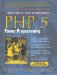# Section 8.4. SimpleXML

### 8.4. SimpleXML

The SimpleXML extension, enabled by default in PHP 5, is the easiest way to work with XML. You don't need to remember a difficult DOM API. You just access the XML through a data structure representation. Here are its four simple rules:

1. Properties denote element iterators.

2. Numeric indices denote elements.

3. Non-numeric indices denote attributes.

By using these four rules, you can access all the data from an XML file.

#### 8.4.1. Creating a SimpleXML Object

You can create a SimpleXML object in any of three ways, as shown in this example:

` <?php   \$sx1 = simplexml_load_file('example.xml'); \$string = <<<XML <?xml version='1.0'?> <html xmlns="http://www.w3.org/1999/xhtml" xml:lang="en" lang="en">   <head>     <title>XML Example</title>   </head>   <body background="bg.png">     <p>       Moved to <a href="http://example.org/">example.org<a>.     </p>     <pre>       foo     </pre>     <p>       Moved to <a href="http://example.org/">example.org</a>.     </p>   </body> </html> XML;   \$sx2 = simplexml_load_string(\$string);   \$sx3 = simplexml_load_dom(new DomDocument()); ?> `

In the first method, simplexml_load_file() opens the specified file and parses it into memory. In the second method, \$string is created and passed to the function simplexml_load_string(). In the third method, simplexml_load_dom() imports a DomDocument created with the DOM functions in PHP. In all three cases, a SimpleXML object is returned. The simplexml_load_dom() function in SimpleXML extension has a brother in the DOM extension, called dom_import_simplexml(). These related functions allow you to share the same XML structure between both extensions. You can, for example, modify simple documents with SimpleXML and more complicated ones with DOM.

#### 8.4.2. Browsing SimpleXML Objects

The first rule is "Properties denote element iterators," which means that you can loop over all <p> tags in the <body>, like this:

` <?php   foreach (\$sx2->body->p as \$p) {   } ?> `

The second states "Numeric indices denote elements," which means that we can access the second <p> tag with

` <?php   \$sx->body->p; ?> `

The third rule is "Non-numeric indexes denote attributes," which means that we can access the background attribute of the body tag with

` <?php   echo \$sx->body['background']; ?> `

The last rule, "String conversion allows access to TEXT data," means we can access all text data from the elements. With the following code, we echo the contents of the second <p> tag (thus combining rules 2 and 4):

` <?php   echo \$sx->body->p; ?> `

However, the output doesn't show Moved to example.org.. Rather, it shows Moved to .. As you can see, accessing TEXT data from a node will not include its child nodes. You can use the asXML() method to include child nodes, but this will also add all the text. Using strip_tags() prevents this. The following example outputs Moved to example.org:

` <?php   echo strip_tags(\$sx->body->p->asXML()) . "\n"; ?> `

If you want to iterate over all child elements of the body node, use the children() method of the SimpleXML element object. The following example iterates over all children of <body>:

` <?php   foreach (\$sx->body->children() as \$element) {    /* do something with the element */   } ?> `

If you want to iterate over all the attributes of an element, the attributes() method is available to you. Let's iterate over all the attributes of the first <a> tag:

` <?php   foreach (\$sx->body->p->a->attributes() as \$attribute) {     echo \$attribute . "\n";   } ?> `

#### 8.4.3. Storing SimpleXML Objects

You can store a changed or manipulated structure or a subnode to disk. You use the asXML() method to do this, which you can call on any SimpleXML object:

` <?php   file_put_contents('filename.xml', \$sx2->asXML()); ?> `PHP 5 Power Programming
ISBN: 013147149X
EAN: 2147483647
Year: 2003
Pages: 240

Similar book on Amazon